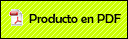# On time-optimal procedure for analog system design

###Miranda Romagnoli, Pedro Amado2004

A. Zemliak, E. Rios, P. Miranda, K. Zemliak, On Time-Optimal Procedure for Analog System Design, Journal of Applied Research and Technology, Vol. 2, No. 1, pp. 32-53, 2004.

Abstract

The process of any analog system design has been formulated on the basis of the control theory application. This approach produces many different design strategies inside the same optimization procedure and allows determining the problem of the optimal design strategy existence from the computer time point of view. Different kinds of system design strategies have been evaluated from the operations number. The general methodology for the analog system design was formulated by means of the optimum control theory. The main equations for this design methodology were elaborated. These equations include the special control functions that are introduced artificially. This approach generalizes the design process and generates an infinite number of the different design strategies. The problem of the optimum design algorithm construction is defined as the minimum-time problem of the control theory. Numerical results of some electronic circuit design demonstrate the efficiency and perspective of the proposed methodology. These examples show that the computer time gain of the optimal design strategy with respect to the traditional design strategy increases when the size and complexity of the system increase. An additional acceleration effect of the design process has been discovered by the analysis of various design strategies with the different initial points. This effect is displayed for all analyzed circuits and it reduces additionally the total computer time for the system design.Fluido magneto reológico bajo perturbaciones magnéticas

Efectos De Una Interacción No Conmutativa En La Radiación De Hawking

Sobre las dimensiones extras espaciales

A Fuzzy Control for Optimizing the Design of Passive Electrical Circuits

A Proposal to Extend Duality in String Theory in Three Dimensions

On time-optimal procedure for analog system design

5D gravitational waves from complexified black rings

Extended T-Duality In Three Dimensions